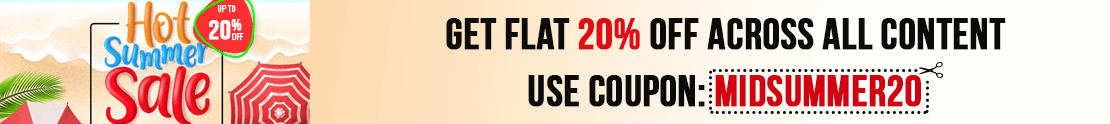# User ForumSubject :IMO    Class : Class 6

## Ans 1:

Class : Class 6

Class : Class 1

(A): Almirah cost him = Cost of Almirah + Amount for cartage + Amount for repair
= 3675 + 172.50 + 64.80
= Rs 3912.30

Subject :IMO    Class : Class 6

Class : Class 1

(A):Direction Umesh is now w.r.t starting point is East
Distance Umesh is from starting point = 20 + 15 = 35m

Subject :IMO    Class : Class 4

Class : Class 1

Option B is the correct answer.

Subject :IMO    Class : Class 4

Class : Class 1

The correct answer is option D.

Class : Class 4

## Ans 3:

Class : Class 5

Subject :IMO    Class : Class 8

Subject :IMO    Class : Class 3

Class : Class 1

(D):

Number of eggs bought per day = 44

Number of days in October = 31

Number of eggs bought in October = 44 x 31 = 1364

Subject :IMO    Class : Class 5

Class : Class 5

Class : Class 6

## Ans 3:

Class : Class 5

Subject :IMO    Class : Class 6

Class : Class 1

(D): L.C.M. of 15, 25, 30 and 45 = 450
450 – 9 = 441.
So, if 9 is added to 441, the number is divisible by all the 4 numbers.

Subject :IMO    Class : Class 3

Class : Class 1

(D):

After interchanging the digits numbers are :
P - 7849, Q - 7859, R - 7889, S - 6349
So, S is the smallest number.